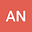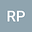Schauder's type of fixed point theorem in locally convex space
••• Andrzej Nowakowski,
• Robert Plebaniak
Andrzej Nowakowski
University of Lodz Faculty of Mathematics and Computer Science

Corresponding Author:andrzej.nowakowski@wmii.uni.lodz.pl

Author ProfileRobert Plebaniak
University of Lodz Faculty of Mathematics and Computer Science
Author Profile## Abstract

We introduce the concept of generalized norm in linear vector spaces which extends the classical norm. Using that generalized norm we provide a generalization of Schauder's type theorem. Next we give some applications of this theorem to find solutions of initial value problems.